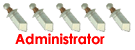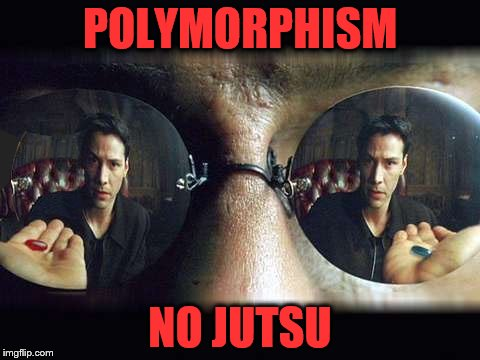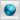battle programmers alliance Would you like to react to this message? Create an account in a few clicks or log in to continue.battle programming a forum for elite programmers with extreme will power to sharpen theire skillsbattle programmers alliance :: battle programming alliance :: chit chat

# polymorphism in JAVA eclipseAuthorMessagePosts : 140
Join date : 2011-08-01Subject: polymorphism in JAVA eclipseWed Jan 31, 2018 9:20 pm polymorphism, exampled via the shape class, and its overridden surface area methode,in the Main class.shape super class : Code: package com.shapes.objects;public class Shape {      public Shape() {      // TODO Auto-generated constructor stub   }   public double surfaceArea() {      return 0;   }} square sub class of shape Code: package com.shapes.objects;public class Square extends Shape {   private double height;   public double getHeight() {      return height;   }   public void setHeight(double height) {      this.height = height;   }   public Square(double height) {      this.height = height;   }   public double surfaceArea() {      return height*height;   }} rectangle sub class of square Code: package com.shapes.objects;public class Rectangle extends Square{   private double width;   public double getWidth() {      return width;   }   public void setWidth(double width) {      this.width = width;   }   public Rectangle(double height, double width) {      super(height);      this.width = width;   }   @Override   public double surfaceArea() {      return super.getHeight()*width;   }   } triangle sub class of shape Code: package com.shapes.objects;public class Triangle extends Shape {   private double height;   private double width;      public Triangle(double height, double width) {      super();      this.height = height;      this.width = width;   }   public double getHeight() {      return height;   }   public void setHeight(double height) {      this.height = height;   }   public double getWidth() {      return width;   }   public void setWidth(double width) {      this.width = width;   }   @Override   public double surfaceArea() {      return (height*width)/2;   }   } circle class extends (sub class of) shape super class: Code: package com.shapes.objects;public class Circle extends Shape {   private double Radius;   public double getRadius() {      return Radius;   }   public void setRadius(double radius) {      Radius = radius;   }      public Circle(double radius) {      super();      Radius = radius;   }   public double surfaceArea() {      return Radius*Radius*3.1415;   }} main class plays around with the classes using polymorphism to refer to all objects as a shapeand using the override methods of the sub classes even though they were declared as the super class : Code: package com.shapes.objects;import java.util.Scanner;import org.w3c.dom.css.Rect;public class Main {   public static void main(String[] args) {      // TODO Auto-generated method stub      Shape sh1 = new Shape();      System.out.println(sh1.surfaceArea());      Rectangle rec1 = new Rectangle(10, 5);      System.out.println(rec1.surfaceArea());      Shape sh2 = rec1;      System.out.println(sh2.surfaceArea());            Shape[] shapeArray = new Shape;            for (int i = 0; i < shapeArray.length; i++) {         System.out.println("enter shape");         Scanner scanner2 = new Scanner(System.in);         String x2 = scanner2.next();         shapeArray[i] = getShape(x2);         System.out.println(shapeArray[i].surfaceArea());      }   }   public static Shape getShape(String form) {      Shape result = null;      Scanner scanner11 = new Scanner(System.in);      switch (form) {      case "square":         System.out.println("enter square side size");         double x1 = scanner11.nextDouble();         return new Square(x1);      case "circle":         System.out.println("enter circle radius");         double Radius1 = scanner11.nextDouble();         return new Circle(Radius1);      case "rectangle":         System.out.println("enter rectangle height");         double height = scanner11.nextDouble();         System.out.println("enter rectangle width");         double width = scanner11.nextDouble();         return new Rectangle(height, width);      case "triangle":         System.out.println("enter triangle height");         double Theight = scanner11.nextDouble();         System.out.println("enter triangle width");         double Twidth = scanner11.nextDouble();         return new Triangle(Theight, Twidth);      default:         break;      }      return result;   }}_________________kurayami no kagipolymorphism in JAVA eclipsePage 1 of 1

Permissions in this forum:You cannot reply to topics in this forumJump to: Select a forum||--battle programming alliance    |--battle programming    |--the LivinGrimoire    |--chit chat